Download Presentation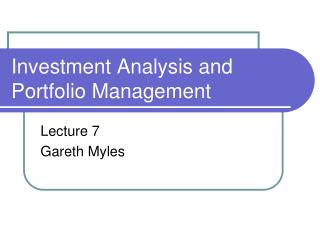Investment Analysis and Portfolio Management

# Investment Analysis and Portfolio Management - PowerPoint PPT Presentation

Investment Analysis and Portfolio Management. Lecture 7 Gareth Myles. The Capital Asset Pricing Model (CAPM). The CAPM is a model of equilibrium in the market for securities.I am the owner, or an agent authorized to act on behalf of the owner, of the copyrighted work described.
Download Presentation## Investment Analysis and Portfolio Management

An Image/Link below is provided (as is) to download presentation

Download Policy: Content on the Website is provided to you AS IS for your information and personal use and may not be sold / licensed / shared on other websites without getting consent from its author.While downloading, if for some reason you are not able to download a presentation, the publisher may have deleted the file from their server.

- - - - - - - - - - - - - - - - - - - - - - - - - - E N D - - - - - - - - - - - - - - - - - - - - - - - - - -
Presentation Transcript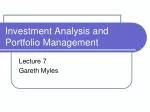### Investment Analysis and Portfolio Management

Lecture 7

Gareth Myles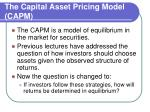The Capital Asset Pricing Model (CAPM)
• The CAPM is a model of equilibrium in the market for securities.
• Previous lectures have addressed the question of how investors should choose assets given the observed structure of returns.
• Now the question is changed to:
• If investors follow these strategies, how will returns be determined in equilibrium?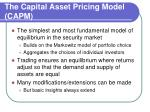The Capital Asset Pricing Model (CAPM)
• The simplest and most fundamental model of equilibrium in the security market
• Builds on the Markowitz model of portfolio choice
• Aggregates the choices of individual investors
• Trading ensures an equilibrium where returns adjust so that the demand and supply of assets are equal
• Many modifications/extensions can be made
• But basic insights always extend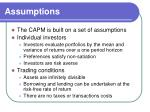Assumptions
• The CAPM is built on a set of assumptions
• Individual investors
• Investors evaluate portfolios by the mean and variance of returns over a one period horizon
• Preferences satisfy non-satiation
• Investors are risk averse
• Assets are infinitely divisible
• Borrowing and lending can be undertaken at the risk-free rate of return
• There are no taxes or transactions costs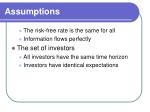Assumptions
• The risk-free rate is the same for all
• Information flows perfectly
• The set of investors
• All investors have the same time horizon
• Investors have identical expectations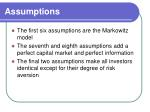Assumptions
• The first six assumptions are the Markowitz model
• The seventh and eighth assumptions add a perfect capital market and perfect information
• The final two assumptions make all investors identical except for their degree of risk aversion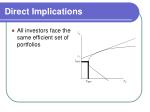Direct Implications
• All investors face the same efficient set of portfolios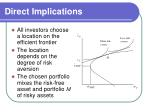Direct Implications
• All investors choose a location on the efficient frontier
• The location depends on the degree of risk aversion
• The chosen portfolio mixes the risk-free asset and portfolio M of risky assets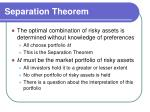Separation Theorem
• The optimal combination of risky assets is determined without knowledge of preferences
• All choose portfolio M
• This is the Separation Theorem
• M must be the market portfolio of risky assets
• All investors hold it to a greater or lesser extent
• No other portfolio of risky assets is held
• There is a question about the interpretation of this portfolio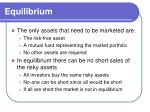Equilibrium
• The only assets that need to be marketed are:
• The risk-free asset
• A mutual fund representing the market portfolio
• No other assets are required
• In equilibrium there can be no short sales of the risky assets
• All investors buy the same risky assets
• No-one can be short since all would be short
• If all are short the market is not in equilibriumEquilibrium
• Equilibrium occurs when the demand for assets matches the supply
• This also applies to the risk-free
• Borrowing must equal lending
• This is achieved by the adjustment of asset prices
• As prices change so do the returns on the assets
• This process generates an equilibrium structure of returnsThe Capital Market Line
• All efficient portfolios must lie on this line
• Slope =
• Equation of the lineInterpretation
• rf is the reward for "time"
• Patience is rewarded
• Investment delays consumption
• is the reward for accepting "risk"
• The market price of risk
• Judged to be equilibrium reward
• Obtained by matching demand to supplySecurity Market Line
• Now consider the implications for individual assets
• Graph covariance against return
• The risk on the market portfolio is
• The covariance of the risk-free asset is zero
• The covariance of the market with the market isSecurity Market Line
• Can mix M and the risk-free asset along the line
• If there was a portfolio above the line all investors would buy it
• No investor would hold one below
• The equation of the line is

MSecurity Market Line
• Define
• The equation of the line becomes
• This is the security market line (SML)Security Market Line
• There is a linear trade-off between risk measured by and return
• In equilibrium all assets and portfolios must have risk-return combinations that lie on this lineMarket Model and CAPM
• Market model uses
• CAPM uses
• is derived from an assumption about the determination of returns
• it is derived from a statistical model
• the index is chosen not specified by any underlying analysis
• is derived from an equilibrium theoryMarket Model and CAPM
• I is usually assumed to be the market index, but in principal could be any index
• M is always the market portfolio
• There is a difference between these
• But they are often used interchangeably
• The market index is taken as an approximation of the market portfolioEstimation of CAPM
• Use the regression equation
• Take the expected value
• The security market line implies
• It also showsCAPM and Pricing
• CAPM also implies the equilibrium asset prices
• The security market line is
• But

where pi(0) is the value of the asset at time 0 and pi(1) is the value at time 1CAPM and Pricing
• So the security market line gives
• This can be rearranged to find
• The price today is related to the expected value at the end of the holding periodCAPM and Project Appraisal
• Consider an investment project
• It requires an investment of p(0) today
• It provides a payment of p(1) in a year
• Should the project be undertaken?
• The answer is yes if the present discounted value (PDV) of the project is positiveCAPM and Project Appraisal
• If both p(0) and p(1) are certain then the risk-free interest rate is used to discount
• The PDV is
• The decision is to accept project ifCAPM and Project Appraisal
• Now assume p(1) is uncertain
• Cannot simply discount at risk-free rate if investors are risk averse
• For example using

will over-value the project

• With risk aversion the project is worth less than its expected returnCAPM and Project Appraisal
• One method to obtain the correct value is to adjust the rate of discount to reflect risk
• But by how much?
• The CAPM pricing rule gives the answer
• The correct PDV of the project is